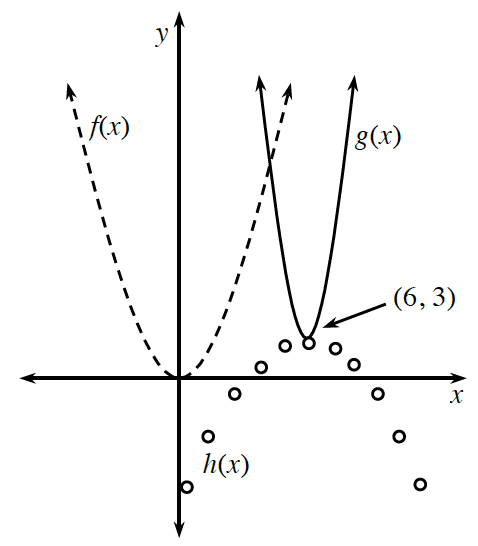### Home > INT2 > Chapter 9 > Lesson 9.1.4 > Problem9-43

9-43.Examine the graph at right that shows three parabolas. The graph of $f(x) = x^2$ is shown as a dashed curve.

1. What is the domain of $f(x)$? The range?

2. Write possible equations for the two other parabolas.

Notice the location of each vertex.

Notice that one is vertically stretched and the other is inverted and vertically compressed.

$g(x)\approx2(x-6)^2+3\\\\h(x)\approx -\frac{1}{2}(\textit{x}-6)^2+3$

3. What is the domain of $g(x)$? The range?

Domain = all real numbers
Range $= y ≥ 3$